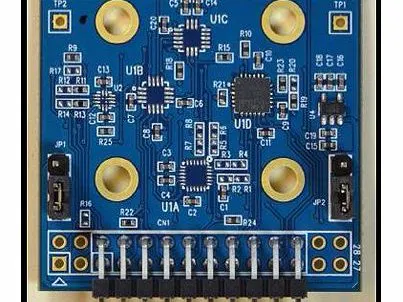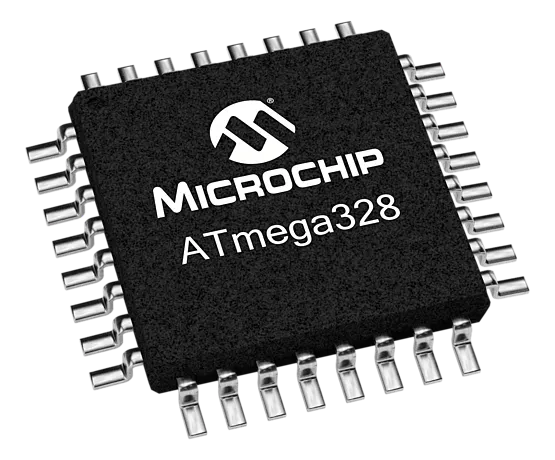# IMU/GPS for Real People

Real talk on GPS and Inertial. No guff. How to realistically use IMUs and GPS modules.

IntermediateFull instructions provided20 hours437## Things used in this project

### Hardware componentsMicrochip ATmega328
×1
 TTL-serial-to-RS232 converter
×1
 LTV-816 optoisolator
×1×1LED (generic)
×1Resistor 1k ohm
×1

## Code

### GPS Decode Example for NEO-8N GPS Module (VB6)

VBScript
Here is some example code using an "exploder" parser that pulls the latitude/longitude/altitude out of a 8N's data stream and puts it into 3 variables (in this case, VB6 text boxes)
```Sub updateposition()

On Error Resume Next
Dim explode(10) As String

s\$ = CStr(Text1.Text)
'find the \$GMRMC sentence
a = InStr(1, s\$, "\$GNRMC", vbTextCompare)

'found it!
If a > 0 Then

'pop off the sentence
b = InStr(a + 1, s\$, vbCrLf, vbTextCompare)
sentence\$ = Mid\$(s\$, a, b - a)

'parse the sentence by exploding it (comma delimited)
a2 = 1
For a = 1 To 10

c = Mid\$(sentence\$, a2, 1)
Do Until c = ","

explode(a) = explode(a) & c

a2 = a2 + 1
c = Mid\$(sentence\$, a2, 1)
Loop

a2 = a2 + 1
Next a

'debug
's3\$ = ""
'For a = 1 To 10: s3\$ = s3\$ & "explode(" & CStr(a) & ")=" & CStr(explode(a)) & vbCrLf: Next a
'MsgBox s3\$

txtLatitude.Text = explode(4) & " " & explode(5)
txtlongitude.Text = explode(6) & " " & explode(7)
txtaltitude.Text = CStr(cdbl2(explode(10)) / 100)

If explode(5) = "N" Then
lat = CDbl(explode(4)) / 100#
Else
lat = -CDbl(explode(4)) / 100#
End If

If explode(7) = "E" Then
lng = CDbl(explode(6)) / 100
Else
lng = -CDbl(explode(6)) / 100#
End If

txtCoordinates.Text = CStr(lat) & " " & CStr(lng)

DoEvents

End If

End Sub
```

## Credits

### Woodward "Woody" Stanford

3 projects • 9 followers